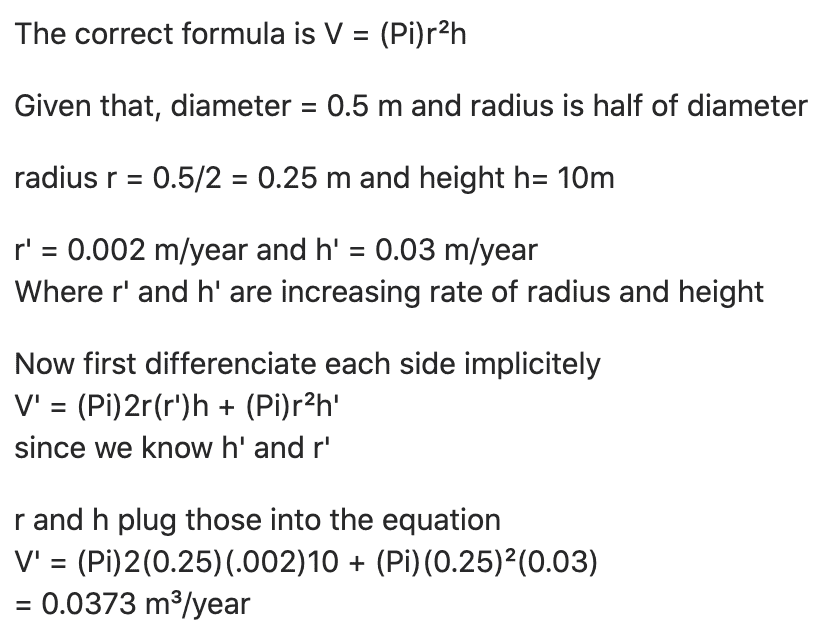# Solving for the speed of volume

Homework Statement:
The trunk of a tree is approximately cylindrical in shape. The radius of the trunk is increasing at a rate of 0.002m/year and the height is increasing at a rate of 0.03 m/year. How fast is the volume of the trunk increasing when the diameter of the tree is 0.5 m and the height of the tree is 10 m?
Relevant Equations:
V = (Pi)r²hIs this correct?